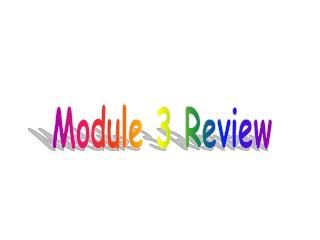# Module 3 Review - PowerPoint PPT PresentationDownload PresentationModule 3 Review

Module 3 ReviewDownload Presentation## Module 3 Review

- - - - - - - - - - - - - - - - - - - - - - - - - - - E N D - - - - - - - - - - - - - - - - - - - - - - - - - - -
##### Presentation Transcript

1. Module 3 Review

2. Section 1 Freshman Total = 375 Spanish French 50 175 100 ? How many are taking Spanish but not French class?

3. Section 1 Freshman Total = 375 Spanish French 50 175 100 ? How many are taking French class but not Spanish?

4. Section 1 Freshman Total = 375 Spanish French 50 175 100 ? How many are taking both French and Spanish?

5. Section 1 Freshman Total = 375 Spanish French 50 175 100 ? How many are taking neither French and Spanish?

6. Section 1 Freshman Total = 375 Spanish French 50 175 100 ? If a new student enrolled into the freshman class and wanted to take both French and Spanish, how would this affect the Venn diagram?

7. Metric Conversion K h Dk m D C M L G 5000 kg = __________metric tons 4200 cm = __________km 9.8 kg = ____________g 6.94 m = ___________ mm 45.5 g = ___________mg

8. Write each fraction as a decimal AND as a percent. 11 25 370 1000 48 200

9. Section 4 Find the range, mean, median, and mode.

10. Write each fraction as a decimal rounded to the hundredth. 2 9 7 4 8 12 11 8

11. Section 5 Find each quotient. 7.25 ÷ 5 = 21.48 ÷ 24 =

12. Use front-end estimation to estimate each sum. 2689 + 4328 = 16.5 + 18.3 = 697 + 878 + 207 =

13. Section 6 Make a stem-and-leaf plot.

14. Find each quotient. Show your long division. 4.824 ÷ .12 = 159.12 ÷ 1.7 =

15. Find the sum or difference. Show your work. 17.49 + 9.064 = 13 + 24.2 = 19 - 4.031 =

16. Section 3 Make a bar graph and a line plot for the data in the table.